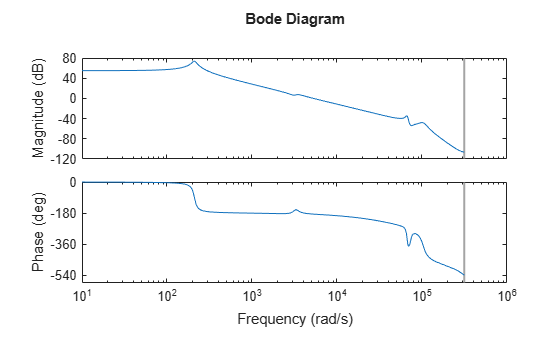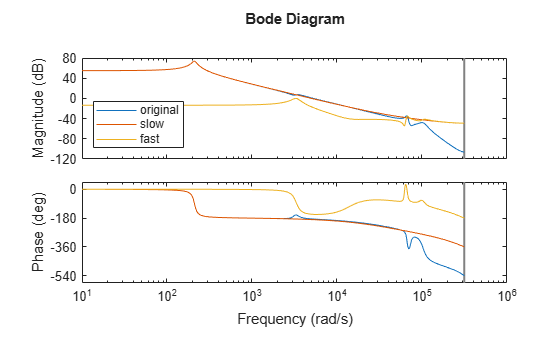Main Content

# freqsep

Slow-fast decomposition

## Syntax

``````[Gs,Gf] = freqsep(G,fcut)``````
``````[Gs,Gf] = freqsep(G,fcut,options)``````

## Description

example

``````[Gs,Gf] = freqsep(G,fcut)``` decomposes a linear dynamic system into slow and fast components around the specified cutoff frequency. The decomposition is such that `G` = `Gs` + `Gf`.```

example

``````[Gs,Gf] = freqsep(G,fcut,options)``` specifies additional options for the decomposition. ```

## Examples

collapse all

Load a dynamic system model.

```load numdemo Pd bode(Pd)````Pd` has four complex poles and one real pole. The Bode plot shows a resonance around 210 rad/s and a higher-frequency resonance below 10,000 rad/s.

Decompose this model around 1000 rad/s to separate these two resonances.

```[Gs,Gf] = freqsep(Pd,10^3); bode(Pd,Gs,Gf) legend('original','slow','fast','Location','Southwest')```The Bode plot shows that the slow component, `Gs`, contains only the lower-frequency resonance. This component also matches the DC gain of the original model. The fast component, `Gf`, contains the higher-frequency resonances and matches the response of the original model at high frequencies. The sum of the two components `Gs+Gf` yields the original model.

Decompose a model into slow and fast components between poles that are closely spaced.

The following system includes a real pole and a complex pair of poles that are all close to s = -2.

`G = zpk(-.5,[-1.9999 -2+1e-4i -2-1e-4i],10);`

Try to decompose the model about 2 rad/s, so that the slow component contains the real pole and the fast component contains the complex pair.

`[Gs,Gf] = freqsep(G,2);`
```Warning: One or more fast modes could not be separated from the slow modes. To force separation, relax the accuracy constraint by increasing the "SepTol" factor (see "freqsepOptions" for details). ```

These poles are too close together for `freqsep` to separate. Increase the relative tolerance to allow the separation.

```options = freqsepOptions('SepTol',5e10); [Gs,Gf] = freqsep(G,2,options);```

Now `freqsep` successfully separates the dynamics.

`slowpole = pole(Gs)`
```slowpole = -1.9999 ```
`fastpole = pole(Gf)`
```fastpole = 2×1 complex -2.0000 + 0.0001i -2.0000 - 0.0001i ```

## Input Arguments

collapse all

Dynamic system to decompose, specified as a numeric LTI model, such as a `ss` or `tf` model.

Cutoff frequency for fast-slow decomposition, specified as a positive scalar. The output `Gs` contains all poles with natural frequency less than `fcut`. The output `Gf` contains all poles with natural frequency greater than or equal to `fcut`.

Options for the decomposition, specified as an options set you create with `freqsepOptions`. Available options include absolute and relative tolerance for accuracy of the decomposed systems.

## Output Arguments

collapse all

Slow dynamics of the decomposed system, returned as a numeric LTI model of the same type as `G`. `Gs` contains all poles of `G` with natural frequency less than `fcut`, and is such that `G` = `Gs` + `Gf`.

Fast dynamics of the decomposed system, returned as a numeric LTI model of the same type as `G`. `Gf` contains all poles of `G` with natural frequency greater than or equal to `fcut`, and is such that `G` = `Gs` + `Gf`.

Model Reducer

## See Also

### Topics

Introduced in R2014a

## Support

#### Learn how to automatically tune PID controller gains

Download code examples